2

# Solutions

Solutions are homogeneous mixtures. Many solutions contain one component called the solvent  in which other components called solutes are dissolved. An aqueous solution is one for which the solvent is water. The concentration of a solution is a measure of the relative amount of solute in a given amount of solution. Concentrations may be measured using various units with one very useful unit being molarity, defined as the number of moles of solute per liter of solution. The solute concentration of a solution may be decreased by adding solvent, a process referred to as dilution. The dilution equation is a simple relation between concentrations and volumes of a solution before and after dilution. Substances that dissolve in water to yield ions are called electrolytes. Electrolytes may be covalent compounds that chemically react with water to produce ions (for example, acids and bases), or they may be ionic compounds that dissociate to yield their constituent cations and anions, when dissolved. Dissolution of an ionic compound is facilitated by ion-dipole attractions between the ions of the compound and the polar water molecules. Soluble ionic substances and strong acids ionize completely and are strong electrolytes while weak acids and bases ionize to only a small extent and are weak electrolytes. Nonelectrolytes are substances that do not produce ions when dissolved in water.

### Learning Objectives

By the end of this section, you will be able to:

• Describe the fundamental properties of solutions
• Calculate solution concentrations using molarity
• Perform dilution calculations using the dilution equation

Preceding sections of this chapter focused on the composition of substances: samples of matter that contain only one type of element or compound. However, mixtures—samples of matter containing two or more substances physically combined—are more commonly encountered in nature than are pure substances. Similar to a pure substance, the relative composition of a mixture plays an important role in determining its properties. The relative amount of oxygen in a planet’s atmosphere determines its ability to sustain aerobic life. The relative amounts of iron, carbon, nickel, and other elements in steel (a mixture known as an “alloy”) determine its physical strength and resistance to corrosion. The relative amount of the active ingredient in a medicine determines its effectiveness in achieving the desired pharmacological effect. The relative amount of sugar in a beverage determines its sweetness (see Figure 2.1). This section will describe one of the most common ways in which the relative compositions of mixtures may be quantified.

Figure 2.1

Sugar is one of many components in the complex mixture known as coffee. The amount of sugar in a given amount of coffee is an important determinant of the beverage’s sweetness. (credit: Jane Whitney)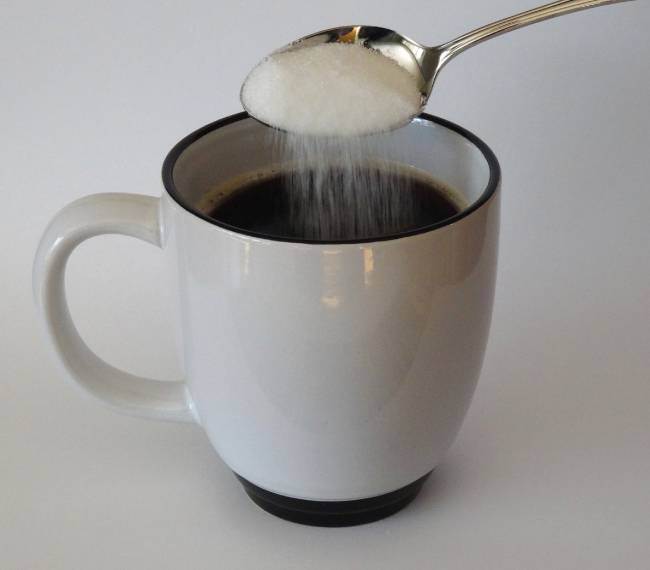## 2.1 Solutions

Solutions have previously been defined as homogeneous mixtures, meaning that the composition of the mixture (and therefore its properties) is uniform throughout its entire volume. Solutions occur frequently in nature and have also been implemented in many forms of manmade technology. A more thorough treatment of solution properties is provided in the chapter on solutions and colloids, but provided here is an introduction to some of the basic properties of solutions.

The relative amount of a given solution component is known as its concentration. Often, though not always, a solution contains one component with a concentration that is significantly greater than that of all other components. This component is called the solvent and may be viewed as the medium in which the other components are dispersed, or dissolved. Solutions in which water is the solvent are, of course very common on our planet. A solution in which water is the solvent is called an aqueous solution.

A solute is a component of a solution that is typically present at a much lower concentration than the solvent. Solute concentrations are often described with qualitative terms such as dilute (of relatively low concentration) and concentrated (of relatively high concentration).

Concentrations may be quantitatively assessed using a wide variety of measurement units, each convenient for particular applications. Molarity (M) is a useful concentration unit for many applications in chemistry. Molarity is defined as the number of moles of solute in exactly 1 liter (1 L) of the solution:

M=mol soluteL solutionM=mol soluteL solution

### EXAMPLE 2.1.1

#### Calculating Molar Concentrations

A 355-mL soft drink sample contains 0.133 mol of sucrose (table sugar). What is the molar concentration of sucrose in the beverage?

#### Solution

Since the molar amount of solute and the volume of solution are both given, the molarity can be calculated using the definition of molarity. Per this definition, the solution volume must be converted from mL to L:
M=mol soluteL solutionmol solute=M×L solutionmol solute=0.375mol sugarL×(10mL×1L1000mL)=0.004mol sugarM=mol soluteL solutionmol solute=M×L solutionmol solute=0.375mol sugarL×(10mL×1L1000mL)=0.004mol sugar

A teaspoon of table sugar contains about 0.01 mol sucrose. What is the molarity of sucrose if a teaspoon of sugar has been dissolved in a cup of tea with a volume of 200 mL?

0.05 M

### EXAMPLE 2.1.2

#### Deriving Moles and Volumes from Molar Concentrations

How much sugar (mol) is contained in a modest sip (~10 mL) of the soft drink from Example 2.1?

#### Solution

Rearrange the definition of molarity to isolate the quantity sought, moles of sugar, then substitute the value for molarity derived in Example 2.1, 0.375 M:
M=mol soluteL solution=0.839MM=0.839mol solute1.00L solutionM=mol soluteL solution=0.839MM=0.839mol solute1.00L solution

What volume (mL) of the sweetened tea described in Example 2.1 contains the same amount of sugar (mol) as 10 mL of the soft drink in this example?

80 mL

### EXAMPLE 2.1.3

#### Calculating Molar Concentrations from the Mass of Solute

Distilled white vinegar (Figure 2.2) is a solution of acetic acid, CH3CO2H, in water. A 0.500-L vinegar solution contains 25.2 g of acetic acid. What is the concentration of the acetic acid solution in units of molarity?

Figure 2.2

Distilled white vinegar is a solution of acetic acid in water.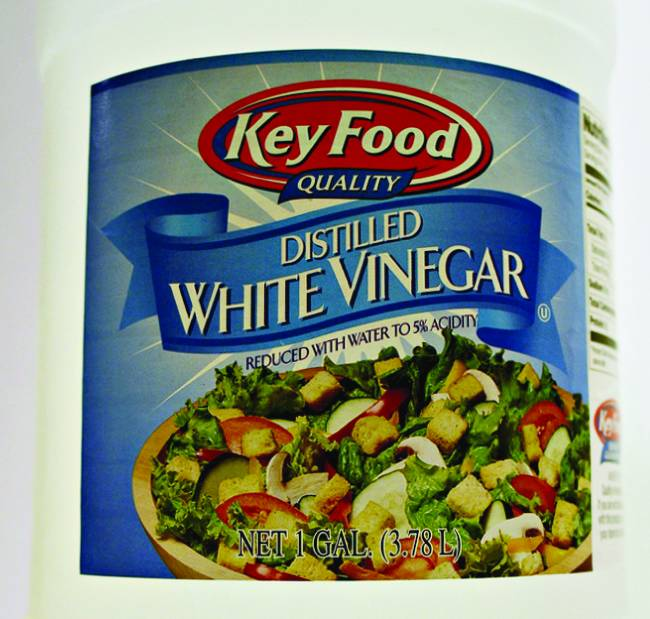#### Solution

As in previous examples, the definition of molarity is the primary equation used to calculate the quantity sought. Since the mass of solute is provided instead of its molar amount, use the solute’s molar mass to obtain the amount of solute in moles:
1.325 mol NaCl×58.44g NaClmol NaCl=77.4g NaCl1.325 mol NaCl×58.44g NaClmol NaCl=77.4g NaCl
mol solute×L solutionmol solute=L solutionmol solute×L solutionmol solute=L solution

Calculate the molarity of 6.52 g of CoCl2  (128.9 g/mol) dissolved in an aqueous solution with a total volume of 75.0 mL.

0.674 M

### EXAMPLE 2.1.4

#### Determining the Mass of Solute in a Given Volume of Solution

How many grams of NaCl are contained in 0.250 L of a 5.30-M solution?

#### Solution

The volume and molarity of the solution are specified, so the amount (mol) of solute is easily computed as demonstrated in Example 2.2:

75.6gCH3CO2H(molCH3CO2H60.05g)(L solution0.839molCH3CO2H)=1.50L solution75.6gCH3CO2H(molCH3CO2H60.05g)(L solution0.839molCH3CO2H)=1.50L solution

Finally, this molar amount is used to derive the mass of NaCl:

n1=M1L1n1=M1L1

How many grams of CaCl2 (110.98 g/mol) are contained in 250.0 mL of a 0.200-M solution of calcium chloride?

5.55 g CaCl2

When performing calculations stepwise, as in Example 2.4, it is important to refrain from rounding any intermediate calculation results, which can lead to rounding errors in the final result. In Example 2.4, the molar amount of NaCl computed in the first step, 1.325 mol, would be properly rounded to 1.32 mol if it were to be reported; however, although the last digit (5) is not significant, it must be retained as a guard digit in the intermediate calculation. If the guard digit had not been retained, the final calculation for the mass of NaCl would have been 77.1 g, a difference of 0.3 g.

In addition to retaining a guard digit for intermediate calculations, rounding errors may also be avoided by performing computations in a single step (see Example 2.5). This eliminates intermediate steps so that only the final result is rounded.

### EXAMPLE 2.1.5

#### Determining the Volume of Solution Containing a Given Mass of Solute

In Example 2.3, the concentration of acetic acid in white vinegar was determined to be 0.839 M. What volume of vinegar contains 75.6 g of acetic acid?

#### Solution

First, use the molar mass to calculate moles of acetic acid from the given mass:
M1L1=M2L2M1L1=M2L2

Then, use the molarity of the solution to calculate the volume of solution containing this molar amount of solute:

C1V1=C2V2C2=C1V1V2C1V1=C2V2C2=C1V1V2

Combining these two steps into one yields:

C1V1=C2V2V2=C1V1C2C1V1=C2V2V2=C1V1C2
C1V1=C2V2V1=C2V2C1C1V1=C2V2V1=C2V2C1

What volume of a 1.50-M KBr solution contains 66.0 g KBr?

0.370 L

## 2.2 Dilution of Solutions

Dilution is the process whereby the concentration of a solution is lessened by the addition of solvent. For example, a glass of iced tea becomes increasingly diluted as the ice melts. The water from the melting ice increases the volume of the solvent (water) and the overall volume of the solution (iced tea), thereby reducing the relative concentrations of the solutes that give the beverage its taste (Figure 2.3).

Figure 2.3

Both solutions contain the same mass of copper nitrate. The solution on the right is more dilute because the copper nitrate is dissolved in more solvent. (credit: Mark Ott)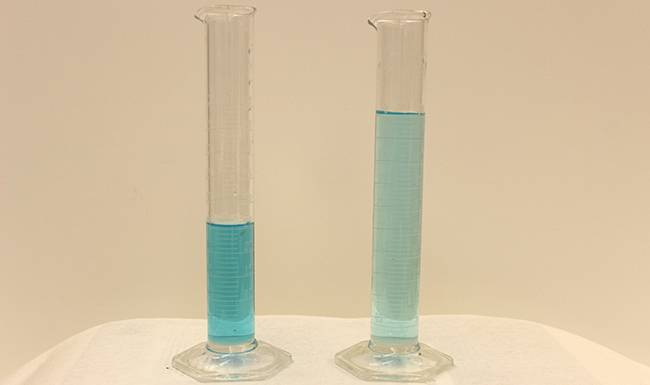Dilution is also a common means of preparing solutions of a desired concentration. By adding solvent to a measured portion of a more concentrated stock solution, a solution of lesser concentration may be prepared. For example, commercial pesticides are typically sold as solutions in which the active ingredients are far more concentrated than is appropriate for their application. Before they can be used on crops the pesticides must be diluted. This is also a very common practice for the preparation of a number of common laboratory reagents.

A simple mathematical relationship can be used to relate the volumes and concentrations of a solution before and after the dilution process. According to the definition of molarity, the number of moles of solute in a solution (n) is equal to the product of the solution’s molarity (M) and its volume in liters (L):

mass percentage=mass of componentmass of solution×100%mass percentage=mass of componentmass of solution×100%

Expressions like these may be written for a solution before and after it is diluted:

××
volume percentage=volume solutevolume solution×100%volume percentage=volume solutevolume solution×100%

where the subscripts “1” and “2” refer to the solution before and after the dilution, respectively. Since the dilution process does not change the amount of solute in the solution, n1 = n2. Thus, these two equations may be set equal to one another:

ppm=mass solutemass solution×106ppmppb=mass solutemass solution×109ppbppm=mass solutemass solution×106ppmppb=mass solutemass solution×109ppb

This relation is commonly referred to as the dilution equation. Although this equation uses molarity as the unit of concentration and liters as the unit of volume, other units of concentration and volume may be used as long as the units properly cancel per the factor-label method. Reflecting this versatility, the dilution equation is often written in the more general form:

ppb=mass solutemass solution×109ppbmass solute=ppb×mass solution109ppbmass solute=15ppb×300mL×1.00gmL109ppb=4.5×10−6gppb=mass solutemass solution×109ppbmass solute=ppb×mass solution109ppbmass solute=15ppb×300mL×1.00gmL109ppb=4.5×10−6g

where C and V are concentration and volume, respectively.

### EXAMPLE 2.2.1

#### Determining the Concentration of a Diluted Solution

If 0.850 L of a 5.00-M solution of copper nitrate, Cu(NO3)2, is diluted to a volume of 1.80 L by the addition of water, what is the molarity of the diluted solution?

#### Solution

The stock concentration, C1, and volume, V1, are provided as well as the volume of the diluted solution, V2. Rearrange the dilution equation to isolate the unknown property, the concentration of the diluted solution, C2:
H2O(l)+H2O(l)⇌H3O+(aq)+OH−(aq)H2O(l)+H2O(l)⇌H3O+(aq)+OH−(aq)

Since the stock solution is being diluted by more than two-fold (volume is increased from 0.85 L to 1.80 L), the diluted solution’s concentration is expected to be less than one-half 5 M. This ballpark estimate will be compared to the calculated result to check for any gross errors in computation (for example, such as an improper substitution of the given quantities). Substituting the given values for the terms on the right side of this equation yields:

C2=0.850L×5.00molL1.80 L=2.36MC2=0.850L×5.00molL1.80 L=2.36M

This result compares well to our ballpark estimate (it’s a bit less than one-half the stock concentration, 5 M).

What is the concentration of the solution that results from diluting 25.0 mL of a 2.04-M solution of CH3OH to 500.0 mL?

0.102 M CH3OH

### EXAMPLE 2.2.2

#### Volume of a Diluted Solution

What volume of 0.12 M HBr can be prepared from 11 mL (0.011 L) of 0.45 M HBr?

#### Solution

Provided are the volume and concentration of a stock solution, V1 and C1, and the concentration of the resultant diluted solution, C2. Find the volume of the diluted solution, V2 by rearranging the dilution equation to isolate V2:
C1V1=C2V2V2=C1V1C2C1V1=C2V2V2=C1V1C2

Since the diluted concentration (0.12 M) is slightly more than one-fourth the original concentration (0.45 M), the volume of the diluted solution is expected to be roughly four times the original volume, or around 44 mL. Substituting the given values and solving for the unknown volume yields:

V2=(0.45M)(0.011L)(0.12M)V2=0.041LV2=(0.45M)(0.011L)(0.12M)V2=0.041L

The volume of the 0.12-M solution is 0.041 L (41 mL). The result is reasonable and compares well with the rough estimate.

A laboratory experiment calls for 0.125 M HNO3. What volume of 0.125 M HNO3 can be prepared from 0.250 L of 1.88 M HNO3?

3.76 L

### EXAMPLE 2.2.3

#### Volume of a Concentrated Solution Needed for Dilution

What volume of 1.59 M KOH is required to prepare 5.00 L of 0.100 M KOH?

#### Solution

Given are the concentration of a stock solution, C1, and the volume and concentration of the resultant diluted solution, V2 and C2. Find the volume of the stock solution, V1 by rearranging the dilution equation to isolate V1:
C1V1=C2V2V1=C2V2C1C1V1=C2V2V1=C2V2C1

Since the concentration of the diluted solution 0.100 M is roughly one-sixteenth that of the stock solution (1.59 M), the volume of the stock solution is expected to be about one-sixteenth that of the diluted solution or around 0.3 liters. Substituting the given values and solving for the unknown volume yields:

V1=(0.100M)(5.00L)1.59MV1=0.314LV1=(0.100M)(5.00L)1.59MV1=0.314L

Thus, 0.314 L of the 1.59-M solution is needed to prepare the desired solution. This result is consistent with the rough estimate.

What volume of a 0.575-M solution of glucose, C6H12O6, can be prepared from 50.00 mL of a 3.00-M glucose solution?

0.261 L

### Learning Objectives

By the end of this section, you will be able to:

• Define the concentration units of mass percentage, volume percentage, mass-volume percentage, parts-per-million (ppm), and parts-per-billion (ppb)
• Perform computations relating a solution’s concentration and its components’ volumes and/or masses using these units

The previous section introduced molarity, a very useful measurement unit for evaluating the concentration of solutions. However, molarity is only one measure of concentration. This section will describe some other units of concentration that are commonly used in various applications, either for convenience or by convention.

## 2.3 Mass Percentage

Earlier in this chapter, percent composition was introduced as a measure of the relative amount of a given element in a compound. Percentages are also commonly used to express the composition of mixtures including solutions. The mass percentage of a solution component is defined as the ratio of the component’s mass to the solution’s mass, expressed as a percentage:

mass percentage=mass of componentmass of solution×100%mass percentage=mass of componentmass of solution×100%

Mass percentage is also referred to by similar names such as percent mass, percent weight, weight/weight percent, and other variations on this theme. The most common symbol for mass percentage is simply the percent sign, %, although more detailed symbols are often used including %mass, %weight, and (w/w)%. Use of these more detailed symbols can prevent confusion of mass percentages with other types of percentages, such as volume percentages (to be discussed later in this section).

Mass percentages are popular concentration units for consumer products. The label of a typical liquid bleach bottle (Figure 2.4) cites the concentration of its active ingredient, sodium hypochlorite (NaOCl), as being 7.4%. A 100.0-g sample of bleach would therefore contain 7.4 g of NaOCl.

Figure 2.4

Liquid bleach is an aqueous solution of sodium hypochlorite (NaOCl). This brand has a concentration of 7.4% NaOCl by mass.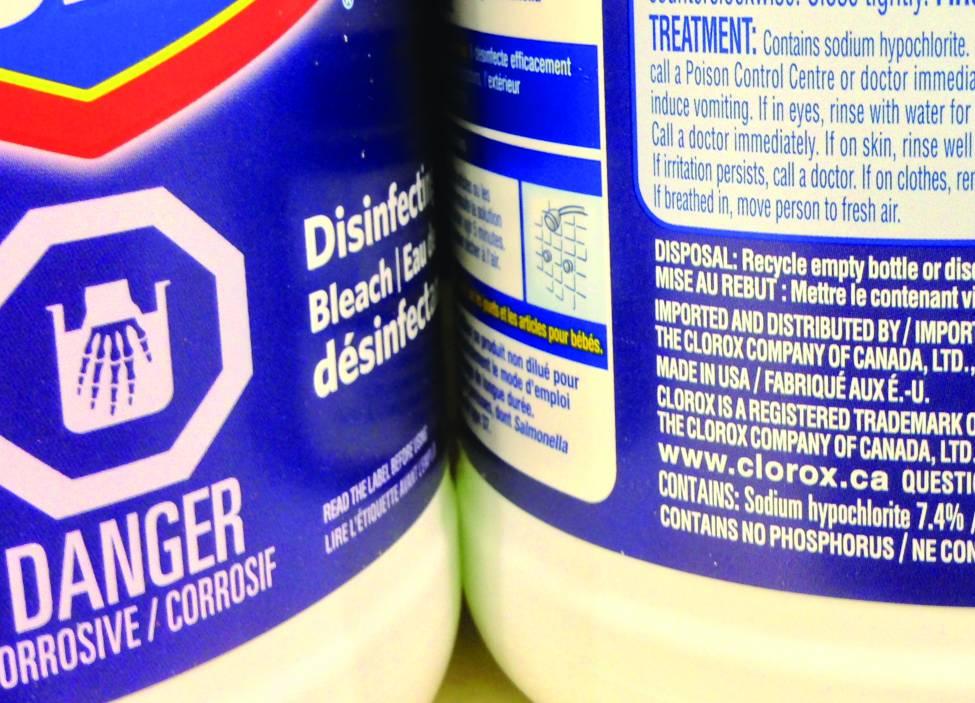### EXAMPLE 2.3.1

#### Calculation of Percent by Mass

A 5.0-g sample of spinal fluid contains 3.75 mg (0.00375 g) of glucose. What is the percent by mass of glucose in spinal fluid?

#### Solution

The spinal fluid sample contains roughly 4 mg of glucose in 5000 mg of fluid, so the mass fraction of glucose should be a bit less than one part in 1000, or about 0.1%. Substituting the given masses into the equation defining mass percentage yields:
%glucose=3.75mg glucose×1g1000mg5.0g spinal fluid=0.075%%glucose=3.75mg glucose×1g1000mg5.0g spinal fluid=0.075%

The computed mass percentage agrees with our rough estimate (it’s a bit less than 0.1%).

Note that while any mass unit may be used to compute a mass percentage (mg, g, kg, oz, and so on), the same unit must be used for both the solute and the solution so that the mass units cancel, yielding a dimensionless ratio. In this case, the solute mass unit in the numerator was converted from mg to g to match the units in the denominator. Alternatively, the spinal fluid mass unit in the denominator could have been converted from g to mg instead. As long as identical mass units are used for both solute and solution, the computed mass percentage will be correct.

A bottle of a tile cleanser contains 135 g of HCl and 775 g of water. What is the percent by mass of HCl in this cleanser?

14.8%

### EXAMPLE 2.3.2

#### Calculations using Mass Percentage

“Concentrated” hydrochloric acid is an aqueous solution of 37.2% HCl that is commonly used as a laboratory reagent. The density of this solution is 1.19 g/mL. What mass of HCl is contained in 0.500 L of this solution?

#### Solution

The HCl concentration is near 40%, so a 100-g portion of this solution would contain about 40 g of HCl. Since the solution density isn’t greatly different from that of water (1 g/mL), a reasonable estimate of the HCl mass in 500 g (0.5 L) of the solution is about five times greater than that in a 100 g portion, or 5 ×× 40 = 200 g. In order to derive the mass of solute in a solution from its mass percentage, the mass of the solution must be known. Using the solution density given, convert the solution’s volume to mass, and then use the given mass percentage to calculate the solute mass. This mathematical approach is outlined in this flowchart:For proper unit cancellation, the 0.500-L volume is converted into 500 mL, and the mass percentage is expressed as a ratio, 37.2 g HCl/g solution:

500 mL solution(1.19g solutionmL solution)(37.2g HCl100g solution)=221g HCl500 mL solution(1.19g solutionmL solution)(37.2g HCl100g solution)=221g HCl

This mass of HCl is consistent with our rough estimate of approximately 200 g.

What volume of concentrated HCl solution contains 125 g of HCl?

282 mL

## 2.4 Volume Percentage

Liquid volumes over a wide range of magnitudes are conveniently measured using common and relatively inexpensive laboratory equipment. The concentration of a solution formed by dissolving a liquid solute in a liquid solvent is therefore often expressed as a volume percentage, %vol or (v/v)%:

volume percentage=volume solutevolume solution×100%volume percentage=volume solutevolume solution×100%

### EXAMPLE 2.4.1

#### Calculations using Volume Percentage

Rubbing alcohol (isopropanol) is usually sold as a 70%vol aqueous solution. If the density of isopropyl alcohol is 0.785 g/mL, how many grams of isopropyl alcohol are present in a 355 mL bottle of rubbing alcohol?

#### Solution

Per the definition of volume percentage, the isopropanol volume is 70% of the total solution volume. Multiplying the isopropanol volume by its density yields the requested mass:
(355mL solution)(70mL isopropyl alcohol100mL solution)(0.785g isopropyl alcohol1mL isopropyl alcohol)=195g isopropyl alchol(355mL solution)(70mL isopropyl alcohol100mL solution)(0.785g isopropyl alcohol1mL isopropyl alcohol)=195g isopropyl alchol

Wine is approximately 12% ethanol (CH3CH2OH) by volume. Ethanol has a molar mass of 46.06 g/mol and a density 0.789 g/mL. How many moles of ethanol are present in a 750-mL bottle of wine?

1.5 mol ethanol

## 2.5 Mass-Volume Percentage

“Mixed” percentage units, derived from the mass of solute and the volume of solution, are popular for certain biochemical and medical applications. A mass-volume percent is a ratio of a solute’s mass to the solution’s volume expressed as a percentage. The specific units used for solute mass and solution volume may vary, depending on the solution. For example, physiological saline solution, used to prepare intravenous fluids, has a concentration of 0.9% mass/volume (m/v), indicating that the composition is 0.9 g of solute per 100 mL of solution. The concentration of glucose in blood (commonly referred to as “blood sugar”) is also typically expressed in terms of a mass-volume ratio. Though not expressed explicitly as a percentage, its concentration is usually given in milligrams of glucose per deciliter (100 mL) of blood (Figure 2.5).

Figure 2.5

“Mixed” mass-volume units are commonly encountered in medical settings. (a) The NaCl concentration of physiological saline is 0.9% (m/v). (b) This device measures glucose levels in a sample of blood. The normal range for glucose concentration in blood (fasting) is around 70–100 mg/dL. (credit a: modification of work by “The National Guard”/Flickr; credit b: modification of work by Biswarup Ganguly)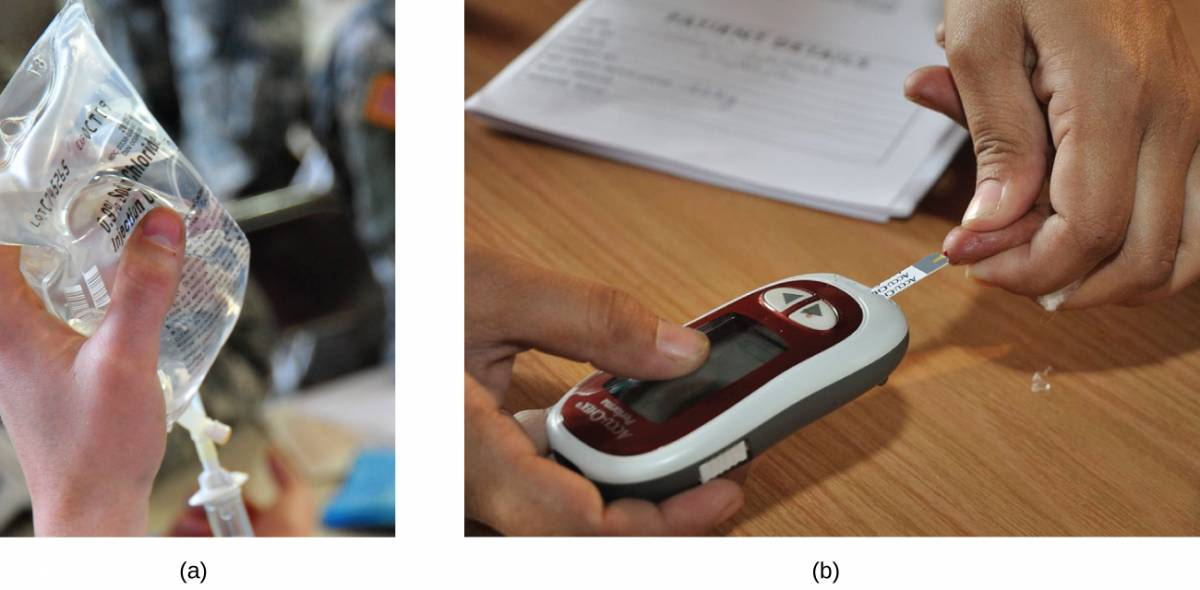## 2.6 Parts per Million and Parts per Billion

Very low solute concentrations are often expressed using appropriately small units such as parts per million (ppm) or parts per billion (ppb). Like percentage (“part per hundred”) units, ppm and ppb may be defined in terms of masses, volumes, or mixed mass-volume units. There are also ppm and ppb units defined with respect to numbers of atoms and molecules.

The mass-based definitions of ppm and ppb are given here:

ppm=mass solutemass solution×106ppmppb=mass solutemass solution×109ppbppm=mass solutemass solution×106ppmppb=mass solutemass solution×109ppb

Both ppm and ppb are convenient units for reporting the concentrations of pollutants and other trace contaminants in water. Concentrations of these contaminants are typically very low in treated and natural waters, and their levels cannot exceed relatively low concentration thresholds without causing adverse effects on health and wildlife. For example, the EPA has identified the maximum safe level of fluoride ion in tap water to be 4 ppm. Inline water filters are designed to reduce the concentration of fluoride and several other trace-level contaminants in tap water (Figure 2.6).

Figure 2.6

(a) In some areas, trace-level concentrations of contaminants can render unfiltered tap water unsafe for drinking and cooking. (b) Inline water filters reduce the concentration of solutes in tap water. (credit a: modification of work by Jenn Durfey; credit b: modification of work by “vastateparkstaff”/Wikimedia commons)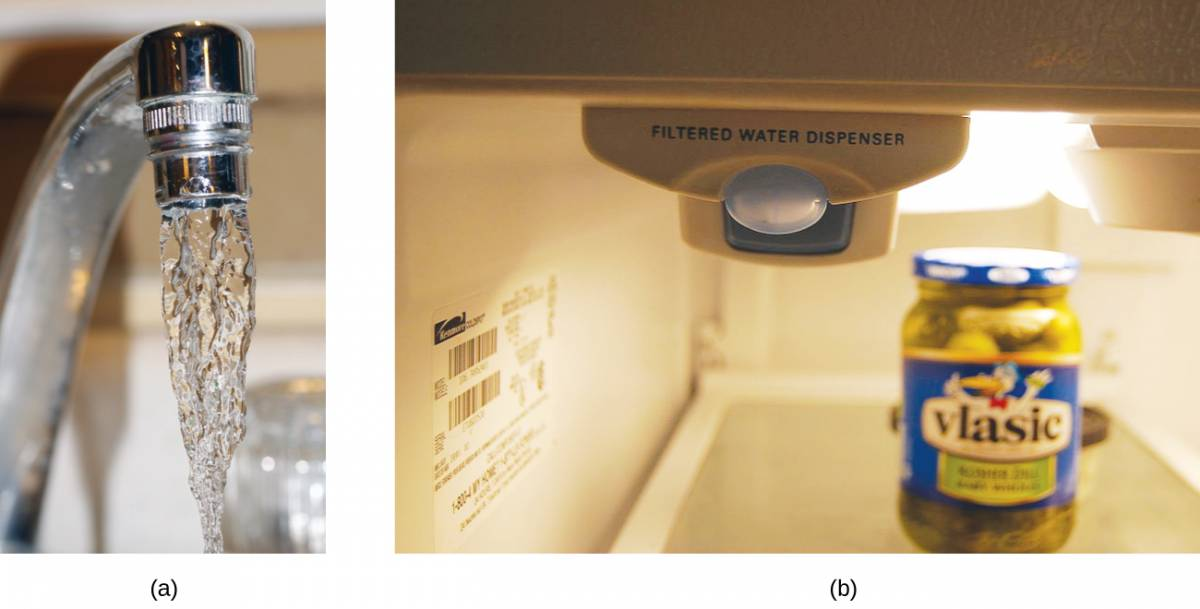### EXAMPLE 2.6.1

#### Calculation of Parts per Million and Parts per Billion Concentrations

According to the EPA, when the concentration of lead in tap water reaches 15 ppb, certain remedial actions must be taken. What is this concentration in ppm? At this concentration, what mass of lead (μg) would be contained in a typical glass of water (300 mL)?

#### Solution

The definitions of the ppm and ppb units may be used to convert the given concentration from ppb to ppm. Comparing these two unit definitions shows that ppm is 1000 times greater than ppb (1 ppm = 103 ppb). Thus:
15ppb×1ppm103ppb=0.015ppm15ppb×1ppm103ppb=0.015ppm

The definition of the ppb unit may be used to calculate the requested mass if the mass of the solution is provided. Since the volume of solution (300 mL) is given, its density must be used to derive the corresponding mass. Assume the density of tap water to be roughly the same as that of pure water (~1.00 g/mL), since the concentrations of any dissolved substances should not be very large. Rearranging the equation defining the ppb unit and substituting the given quantities yields:

ppb=mass solutemass solution×109ppbmass solute=ppb×mass solution109ppbmass solute=15ppb×300mL×1.00gmL109ppb=4.5×10−6gppb=mass solutemass solution×109ppbmass solute=ppb×mass solution109ppbmass solute=15ppb×300mL×1.00gmL109ppb=4.5×10−6g

Finally, convert this mass to the requested unit of micrograms:

μμμμ4.5×10−6g×1μg10−6g=4.5μg4.5×10−6g×1μg10−6g=4.5μg

A 50.0-g sample of industrial wastewater was determined to contain 0.48 mg of mercury. Express the mercury concentration of the wastewater in ppm and ppb units.

9.6 ppm, 9600 ppb

## 2.7 Electrolytes

### Learning Objectives

By the end of this section, you will be able to:

• Define and give examples of electrolytes
• Distinguish between the physical and chemical changes that accompany dissolution of ionic and covalent electrolytes
• Relate electrolyte strength to solute-solvent attractive forces

When some substances are dissolved in water, they undergo either a physical or a chemical change that yields ions in solution. These substances constitute an important class of compounds called electrolytes. Substances that do not yield ions when dissolved are called nonelectrolytes. If the physical or chemical process that generates the ions is essentially 100% efficient (all of the dissolved compound yields ions), then the substance is known as a strong electrolyte. If only a relatively small fraction of the dissolved substance undergoes the ion-producing process, it is called a weak electrolyte.

Substances may be identified as strong, weak, or nonelectrolytes by measuring the electrical conductance of an aqueous solution containing the substance. To conduct electricity, a substance must contain freely mobile, charged species. Most familiar is the conduction of electricity through metallic wires, in which case the mobile, charged entities are electrons. Solutions may also conduct electricity if they contain dissolved ions, with conductivity increasing as ion concentration increases. Applying a voltage to electrodes immersed in a solution permits assessment of the relative concentration of dissolved ions, either quantitatively, by measuring the electrical current flow, or qualitatively, by observing the brightness of a light bulb included in the circuit (Figure 2.7).

Figure 2.7

Solutions of nonelectrolytes such as ethanol do not contain dissolved ions and cannot conduct electricity. Solutions of electrolytes contain ions that permit the passage of electricity. The conductivity of an electrolyte solution is related to the strength of the electrolyte.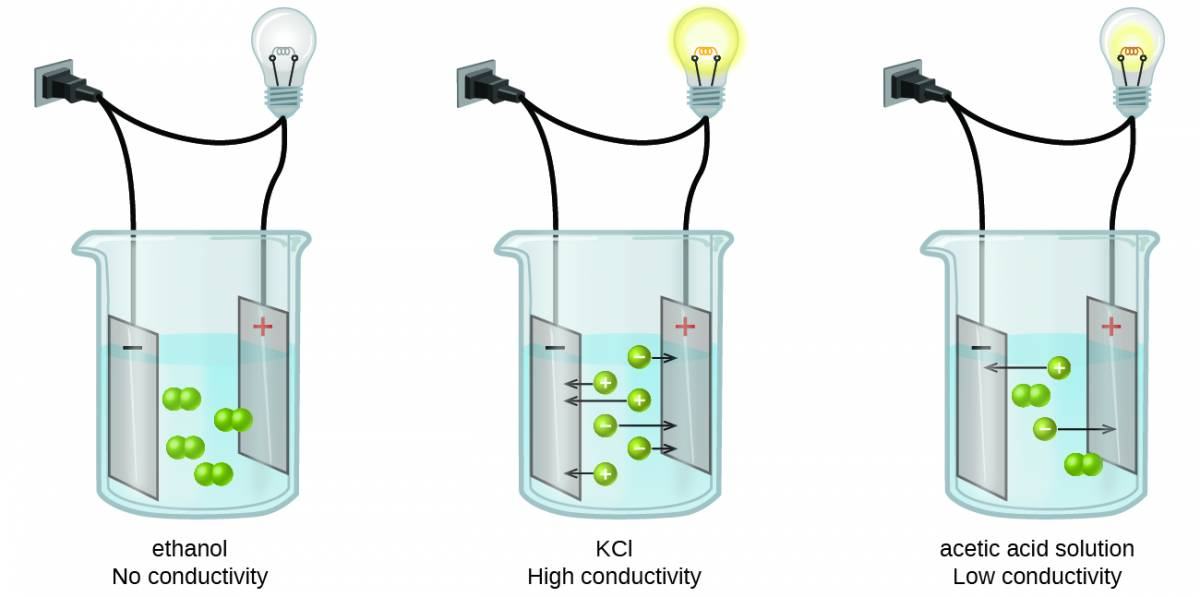## 2.8 Ionic Electrolytes

Water and other polar molecules are attracted to ions, as shown in Figure 2.8. The electrostatic attraction between an ion and a molecule with a dipole is called an ion-dipole attraction. These attractions play an important role in the dissolution of ionic compounds in water.

Figure 2.8

As potassium chloride (KCl) dissolves in water, the ions are hydrated. The polar water molecules are attracted by the charges on the K+ and Cl− ions. Water molecules in front of and behind the ions are not shown.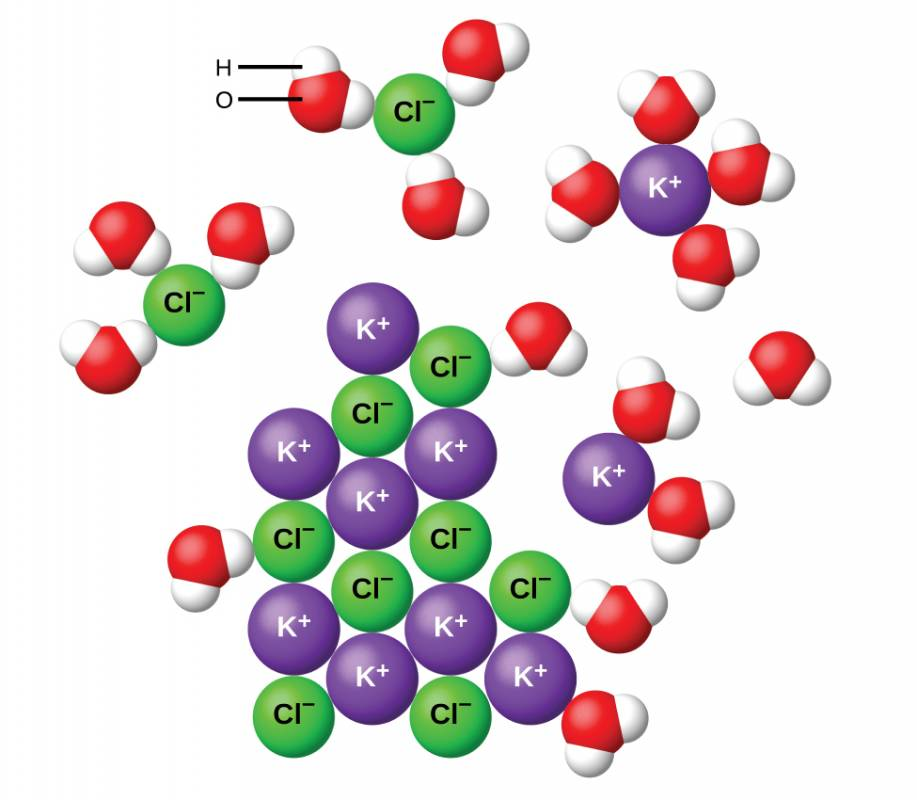When ionic compounds dissolve in water, the ions in the solid separate and disperse uniformly throughout the solution because water molecules surround and solvate the ions, reducing the strong electrostatic forces between them. This process represents a physical change known as dissociation. Under most conditions, ionic compounds will dissociate nearly completely when dissolved, and so they are classified as strong electrolytes. Even sparingly, soluble ionic compounds are strong electrolytes, since the small amount that does dissolve will dissociate completely.

Consider what happens at the microscopic level when solid KCl is added to water. Ion-dipole forces attract the positive (hydrogen) end of the polar water molecules to the negative chloride ions at the surface of the solid, and they attract the negative (oxygen) ends to the positive potassium ions. The water molecules surround individual K+ and Cl− ions, reducing the strong interionic forces that bind the ions together and letting them move off into solution as solvated ions, as Figure 2.8 shows. Overcoming the electrostatic attraction permits the independent motion of each hydrated ion in a dilute solution as the ions transition from fixed positions in the undissolved compound to widely dispersed, solvated ions in solution.

## 2.9 Covalent Electrolytes

Pure water is an extremely poor conductor of electricity because it is only very slightly ionized—only about two out of every 1 billion molecules ionize at 25 °C. Water ionizes when one molecule of water gives up a proton (H+ ion) to another molecule of water, yielding hydronium and hydroxide ions.

H2O(l)+H2O(l)⇌H3O+(aq)+OH−(aq)H2O(l)+H2O(l)⇌H3O+(aq)+OH−(aq)

In some cases, solutions prepared from covalent compounds conduct electricity because the solute molecules react chemically with the solvent to produce ions. For example, pure hydrogen chloride is a gas consisting of covalent HCl molecules. This gas contains no ions. However, an aqueous solution of HCl is a very good conductor, indicating that an appreciable concentration of ions exists within the solution.

Because HCl is an acid, its molecules react with water, transferring H+ ions to form hydronium ions (H3O+) and chloride ions (Cl−):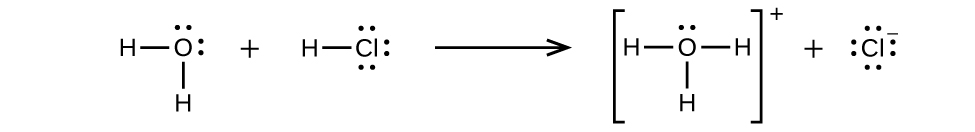This reaction is essentially 100% complete for HCl (i.e., it is a strong acid and, consequently, a strong electrolyte). Likewise, weak acids and bases that only react partially generate relatively low concentrations of ions when dissolved in water and are classified as weak electrolytes. The reader may wish to review the discussion of strong and weak acids provided in the earlier chapter of this text on reaction classes and stoichiometry.

## Files

Previous Citation(s)
Flowers, P., et al. (2019). Chemistry: Atoms First 2e. https://openstax.org/details/books/chemistry-atoms-first-2e (6.3-6.4, 11.2)